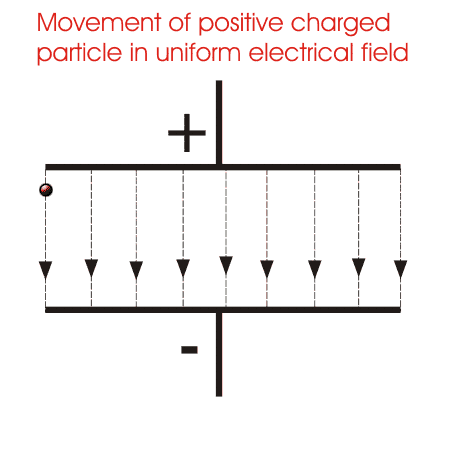# We know that electric potential is quantity. But we measure it only in terms of difference.. why? What exactly is electric potential?

+1 vote
1.2k views
in JEE
edited

We know that electric potential is quantity. But we measure it only in terms of difference.. why? What exactly is electric potential?

by (29.3k points)

Electric Potential

In an electric field, when an object is moved against the electric field it gains some amount of energy which is defined as the electric potential energy.

The magnitude of this energy depends on the amount of work done on the object in moving the object from one point to another against the electric field.  Electric potential energy is defined as the total amount of work done by an external agent in moving a charge from an arbitrarily chosen reference point, which we usually take as infinity ( as it is assumed that electrical potential of a charge at infinity is zero) to that position without any acceleration. For any charge,

Electric potential energy is defined as the total amount of work done by an external agent in moving a charge from an arbitrarily chosen reference point, which we usually take as infinity ( as it is assumed that electrical potential of a charge at infinity is zero) to that position without any acceleration. For any charge, electric potential is obtained by dividing the electric potential energy by the quantity of charge.